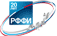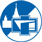Российский фонд
фундаментальных
исследованийФизический факультет
МГУ им. М.В.Ломоносова

# Прикл. мех. 2020. 56, № 1

Гузь А.Н., Багно А.М. «Влияние начальных напряжений на квазилэмбовские моды в гидроупругих волноводах» Прикладная механика, 56, № 1, с. 4-22 (2020)

The problem of quasi-Lamb waves propagation in a pre-deformed compressible elastic layer that interacts with a half-space of an ideal compressible fluid and in a pre-deformed compressible elastic half-space that interacts with a layer of an ideal compressible fluids is studied. The study is carried out basing on the three-dimensional linearized equations of the theory of elasticity of finite deformations for the compressible elastic solid and the three-dimensional linearized Euler equations for the ideal compressible fluid. A problem statement and an approach, based on the representations of general solutions of the linearized equations for elastic solid and fluid are used. The dispersion equations, which describe a propagation of quasi-Lamb waves in the hydroelastic systems are obtained in the wide frequency range. An effect of the initial stresses as well as the thickness of elastic layer and layer of ideal compressible fluid on the phase velocities of quasi-Lamb modes are analyzed. A criterion of existence of the quasi-Lamb waves in the hydroelastic waveguides is proposed. The developed approach and the findings make it possible to establish the limits of applicability of the models of wave processes, based on different versions of the theory of small initial deformations, as well on the classical theory of elasticity. The numerical results are presented in the form of graphs, and their analysis is given.

Прикладная механика, 56, № 1, с. 4-22 (2020) | Рубрика: 04.15

Петрищев О.Н., Романюк М.И. «Возбуждение пространственно развитых волн Лэмба системой объемных и поверхностных нагрузок (Часть 2)» Прикладная механика, 56, № 1, с. 105-127 (2020)

A problem of excitation of spatially developed Lamb waves by a system of volume and surface loads is formulated and completely analytically solved. With the application of the direct and inverse integral Hankel transform, the relations are obtained for determining the amplitude factors of the radially propagating non-axisymmetric Lamb waves, that are excited by a system of volume and surface loads in an arbitrary region of an isotropic elastic layer. The relations are obtained for calculating the components of the displacement vector of material particles of the elastic layer in the far field.

Прикладная механика, 56, № 1, с. 105-127 (2020) | Рубрика: 04.11